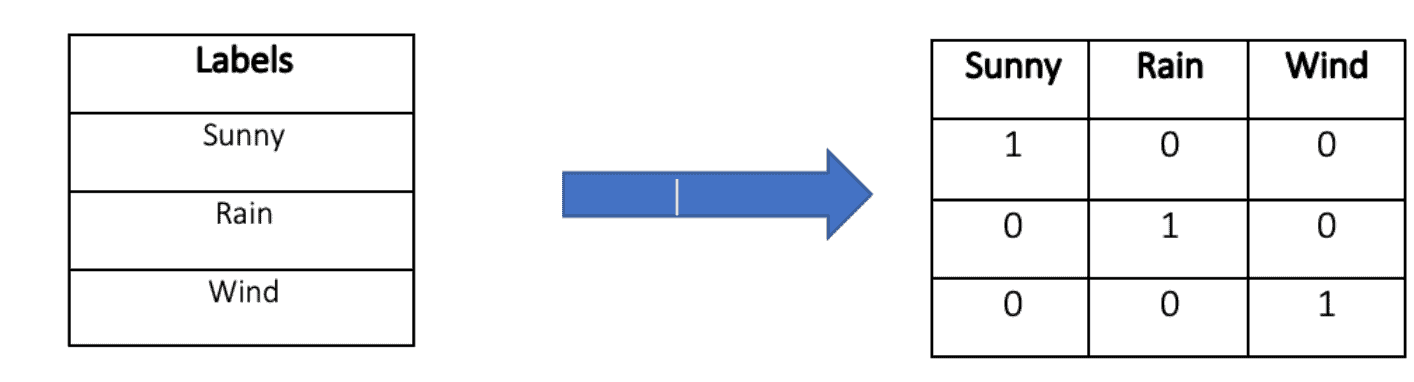## 1. Introduction

Feature engineering boosts the accuracy of machine-learning models by producing new variables in data.

In this tutorial, we’ll look into a particular type of feature engineering we call one-hot encoding.

## 2. Why Do We Encode Data?

We use one-hot encoding when our data contain nominal (categorical) features. By definition, that means that possible values of those features cannot be ordered. For example, in a gender column, we can’t order male and female as female > male or male > female, as that would bias algorithms. For that reason, we can’t assign numerical values to categories, e.g.,and, as that would also imply an order.

Additionally, some machine-learning algorithms expect the input data to contain only numbers, not strings (categories). However, many datasets have at least one categorical variable.

## 3. What Is One-Hot Encoding?

In one-hot encoding, we convert categorical data to multidimensional binary vectors.

The number of dimensions corresponds to the number of categories, and each category gets its dimension. Then, we encode each category by mapping it to a vector in which the element corresponding to the category’s dimension is 1, and the rest are 0, hence the name.

For example, let’s suppose the categorical variable denotes weather prediction and has three categories: sunny, rain, and wind. Then, the encoding can be sunny = [1,0,0], rain = [0,1,0], wind = [0,0,1]:### 3.1. Example

Let’s say we have a Pokemon dataset:Two features are categorical. The Name column acts as the id, so we’ll disregard it when training machine-learning models. However, the Type column contains information that is relevant to learning tasks.

To use it, we apply one-hot encoding. As there are three categories, the vectors will have three dimensions. In each dataset row, we replace the Type category with an encoded vector that has 1 in the position corresponding to the category and contains zeroes in the other two dimensions:This way, we enlarge our data by adding new columns, one per category. We assign descriptive names to the new dummy columns to better navigate the processed dataset.

### 3.2. Dimensionality

If we have a categorical column with a lot of categories, one-hot encoding will add an excessive number of new features. That will take a lot of space and make learning algorithms slow.

To address that issue, we can find the topmost frequent categories and encode only them. For the rest, we create a special column “other” or ignore them. The exact choice ofdepends on our processing power. In practice, we usually go with 10 or 20.

This issue can also occur if we have multiple categorical variables that, in total, produce too many new columns.